Feedback

# Understand different types of strain

### This is an excerpt from Biomechanics of Sport and Exercise 4th Edition With Web Resource-Loose-Leaf Edition by Peter M. McGinnis.

Objects deform when they are subjected to external forces. These deformations may be large or small, depending on the nature of the material and the stresses involved. Strain is the quantification of the deformation of a material. Linear strain occurs as a result of a change in the object's length. Linear strain is produced by compressive or tensile stresses. Shear strain occurs as a result of a change in the orientation of the object's molecules.

### Linear Strain

When the loading of an object causes tensile or compressive stress within the object, some change in length accompanies this stress. This deformation can be measured in absolute terms that describe the change in length of the object as a result of the loading; for example, the rubber band stretched 10 cm or the intervertebral disc compressed 2 mm. The deformation may also be described in relative terms as a proportion of the change in length (the difference between the undeformed and the deformed lengths) divided by the original length (the undeformed length). This relative deformation measure is called linear strain. Mathematically, linear strain is defined as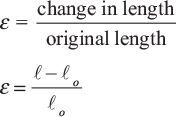where

ε = linear strain,

= stretched length,

o = original, undeformed length, and

o = change in length.

Try self-experiment 9.4.

### Self-Experiment 9.4

Measuring Strain in a Stretched Rubber Band

Take a long rubber band and lay it, unstretched, alongside a ruler. Use a pen to make a mark on it about 1 cm from the left end. Align this mark with zero on the ruler and then make another mark on the rubber band 5 cm from the first mark. Make smaller marks at every 5 mm interval between the zero and 5 cm marks. Now, hook the left end of the rubber band over the zero end of the ruler and pull the slack out of the rubber band. Measure the length of the unstretched rubber band by noting where the right end of the rubber band lines up with the ruler. Now, pull on the right end of the rubber band and stretch it until it is twice its original length. How far apart are the original zero and 5 cm marks on the stretched rubber band? How far apart are any two adjacent 5 mm marks? The end marks are about twice as far apart or 10 cm apart, and the 5 mm marks are now spaced 10 mm apart.

Let's determine the strain in the stretched rubber band in self-experiment 9.4. The distance between the end marks on the rubber band was 5 cm in the unstretched condition and 10 cm after stretching. The absolute deformation of this 5 cm section of the rubber band was

10 cm – 5 cm = 5 cm.

The relative deformation or strain would be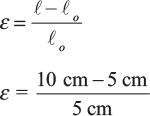ε = 1 cm/cm

or

ε = 100%

Now look at any 5 mm section of the rubber band. Each of these has stretched to 10 mm long, so the strain in every 5 mm section is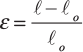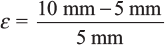ε = 1 mm/mm

or

ε = 100%

The strain between any two adjacent 5 mm marks on the rubber band is also 100%. This makes sense; because the tensile stress is uniform throughout the rubber band, it follows that the strain would be also.

You may have noticed that we have reported strain in centimeters per centimeter, millimeters per millimeter, and as a percentage. Actually, strain is a dimensionless quantity because it is a ratio of length to length. So, centimeters per centimeter is the same as millimeters per millimeter is the same as inches per inch, because the units cancel out. Usually, strain is reported as a percentage, which seems to be more useful. In that case, the ratio is multiplied by 100% to get the percentage of strain. In the preceding example, 1 cm/cm is the same as 1 mm/mm because each equals 100%. Most biological materials are not as elastic as a rubber band, and rupture or failure will result at strains much, much less than 100%.

### Sample Problem 9.2

A sample of biological material is loaded into a material-testing machine. The material is 2 cm long in its unloaded state. A 6000 N tensile force is applied to the material, and it stretches to a length of 2.0004 cm as a result of this force. What is the strain in the specimen when it is stretched this much?

Solution:

Step 1: Identify the known quantities.

= 2.0004 cm

o = 2.0 cm

Step 2: Identify the unknown variable to solve for.

ε = ?

Step 3: Search for an equation with the known and unknown variables. Let's try equation 9.4.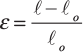Step 4: Substitute the known quantities and solve for the unknown variable.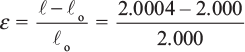ε = 0.0002 = 0.02%

### Shear Strain

Linear strain occurs with a change in length as a result of molecules being pulled apart or pushed together. Shear strain occurs with a change in orientation of adjacent molecules as a result of these molecules slipping past each other. Figure 9.14 graphically illustrates shear strain.

Shear strain is measured as follows. Imagine a line perpendicular to the analysis plane through the object at the location of interest. In two dimensions, this line and the line of the analysis plane form a right angle when the object is undeformed. But, when a shear load is applied to the object, this angle changes. The change in the angle (θ) is the measure of shear strain in the direction of interest. Shear strain is abbreviated with the Greek letter λ (lambda) and is measured in radians.

### Poisson's Ratio

Consider the rubber band again. As you stretched it, what happened to the width of the rubber band? Its width became narrower as it was stretched in length. Consider a rubber ball loaded in compression. The diameter of the ball shortens in the direction of the compressive load, but what about the diameter in the lateral direction? When the rubber ball is compressed, it becomes wider in the lateral direction while shortening in the direction of the compressive load. This is called the Poisson effect, after S.D. Poisson, a French scientist who investigated this phenomenon in the 1820s. A specific ratio of strain in the axial direction to strain in the transverse direction exists for each different type of material. This ratio is called Poisson's ratio. Values of Poisson's ratio can be as low as 0.1 and as high as 0.5, but for most materials they are between 0.25 and 0.35.

The behavior of intervertebral discs provides a good example of the Poisson effect. During the day, your intervertebral discs are loaded in compression (unless you are lying down all day). This compressive load shortens the vertical dimension of the discs, but laterally, the discs bulge out. Under extremely large compressive loads, a disc may bulge too much and rupture.